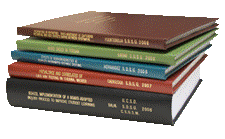Archival Master’s Theses from the University of Nebraska-Lincoln

Thesis

1-17-1967

Citation

Thesis (M.S.)—University of Nebraska—Lincoln, 1967. Department of Engineering Mechanics.

Copyright 1967, the author. Used by permission.

Abstract

In the motion analysis of the coupler points of a four-bar mechanism, one can achieve reproductive results graphically for the displacement, but it is very time consuming. However, when the velocity and acceleration are determined graphically, the results are not very accurate. Rather than obtaining reproducible results one often gets an average value with a plus or minus around this value. Reproducible results are always received for the analysis of the displacement, velocity and acceleration of a coupler point using a digital computer. Since the computer, under normal conditions, stores a number which may have up to eight decimal places, one can be certain that the results will be accurate to as many significant figures as the input numbers are.

As was mentioned earlier in plotting the center-point curve, a complete curve can be plotted graphically from one opposite pole quadrilateral. With four specified positions of a body, there are three distinct opposite pole quadrilaterals and therefore the complete center-point curve can be plotted three times. When this is done graphically, it is very difficult to achieve on curve, rather, the results are more likely to be a “band” or three distinct curves which are very close. This is significant because for the included example in the appendix one opposite side was 20 units long and yet the computer solution resulted in one curve for three plots.

The program as presented may very well be improved upon by changing a part or parts of them. Although the center-point curve can be plotted from one quadrilateral, for this thesis results can be received from all three quadrilaterals. Since the complete curve can be plotted from one quadrilateral, two quadrilaterals could be eliminated from the program in order to save compiling time. The center-point curve results are not capable of being plotted by a computer curve plotter as they appear in this program; however, with a few changes one could adapt this program to a curve plotter.